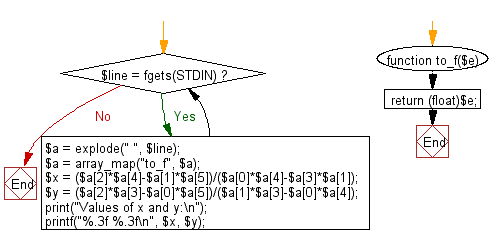﻿ PHP Exercise: Print the values of x, y where a, b, c, d, e and f are specified - w3resource

# PHP Exercises: Print the values of x, y where a, b, c, d, e and f are specified

## PHP: Exercise-49 with Solution

Write a PHP program which solve the equation:
ax+by=c
dx+ey=f
Print the values of x, y where a, b, c, d, e and f are given.

Input:
a,b,c,d,e,f separated by a single space.
(-1,000 ≤ a,b,c,d,e,f ≤ 1,000)

Sample Solution: -

PHP Code:

``````<?php
function to_f(\$e) {
return (float)\$e;
}
while(\$line = fgets(STDIN)) {
\$a = explode(" ", \$line);
\$a = array_map("to_f", \$a);
\$x = (\$a*\$a-\$a*\$a)/(\$a*\$a-\$a*\$a);
\$y = (\$a*\$a-\$a*\$a)/(\$a*\$a-\$a*\$a);
print("Values of x and y:\n");
printf("%.3f %.3f\n", \$x, \$y);
}
?>
```
```

Sample Output:

```Values of x and y:
-1.684 2.737
```

Flowchart:PHP Code Editor:

Have another way to solve this solution? Contribute your code (and comments) through Disqus.

What is the difficulty level of this exercise?

Test your Programming skills with w3resource's quiz.

﻿

## PHP: Tips of the Day

Returns all elements in an array except for the first one

Example:

```<?php
function tips_tail(\$items)
{
return count(\$items) > 1 ? array_slice(\$items, 1) : \$items;
}

print_r(tips_tail([1, 5, 7]));
?>
```

Output:

```Array
(
 => 5
 => 7
)
```